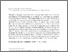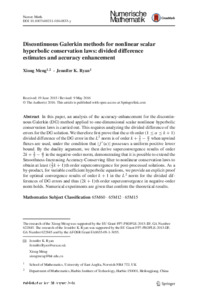# Discontinuous Galerkin methods for nonlinear scalar hyperbolic conservation laws: divided difference estimates and accuracy enhancement

Meng, Xiong and Ryan, Jennifer (2017) Discontinuous Galerkin methods for nonlinear scalar hyperbolic conservation laws: divided difference estimates and accuracy enhancement. Numerische Mathematik, 136 (1). 27–73. ISSN 0029-599XPreview PDF (article_NumerischeMathematik) - Published Version Download (1MB) | Preview

## Abstract

In this paper, an analysis of the accuracy-enhancement for the discontinuous Galerkin (DG) method applied to one-dimensional scalar nonlinear hyperbolic conservation laws is carried out. This requires analyzing the divided difference of the errors for the DG solution. We therefore first prove that the alpha-th order (1 <= \alpha <= k+1) divided difference of the DG error in the L2-norm is of order k+(3-alpha)/2 when upwind fluxes are used, under the condition that |f'(u)| possesses a uniform positive lower bound. By the duality argument, we then derive superconvergence results of order k+(3-alpha)/2 in the negative-order norm, demonstrating that it is possible to extend the Smoothness-Increasing Accuracy-Conserving filter to nonlinear conservation laws to obtain at least (3k/2+1)th order superconvergence for post-processed solutions. As a by-product, for variable coefficient hyperbolic equations, we provide an explicit proof for optimal convergence results of order k+1 in the L2-norm for the divided differences of DG errors and thus (2k+1)th order superconvergence in negative-order norm holds. Numerical experiments are given that confirm the theoretical results.View Item